In the given diagram ACB we just need to draw a free body.  Here, x, y  and P and the angle are known. In addition, the weight of the bar is considered as negligible and thus it is neglected.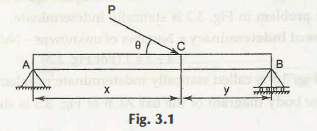If we make it a perfect diagram with free body, then it would be just like the following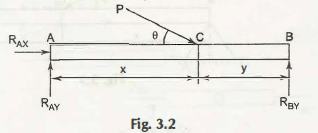What are the unknown numbers here? These are 3 numbers as RBy, RAX and RAy.

Now, the number of equations available are –

Σ M = 0

Σ fy = 0

Σ fx = 0

These are also aviable in 3.

It means the number of unknowns = Number of equations available.

These kind of problems are known as statically determinate problems. The exact problem is prvded in the first figure.

To make the solution ins a proper way the following two diagrams are important to understand –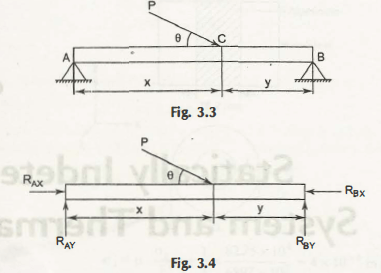Here the number of known quantities is 4 as P, x, θ and y. The weight is neglected.

Now, the number of unknowns are RAx, RBy, RAy‘, RBx = There are 4 unknowns for this

The number of equations = 3

These 3 equations are – Σ fx = 0, Σ fy = 0 and Σ M = 0

Here, if you compare

Number of unknowns > number of equation availability

This kind of problem is known as statically indeterminate.

Now, Degree of Indeterminacy

= Number of unknowns – Number of equations available

= 4 – 3

= 1

It indicates that Degree of Indeterminacy is 1.

It can also be said as degree one of the statically indeterminate problem.

The following diagram shows some other problem as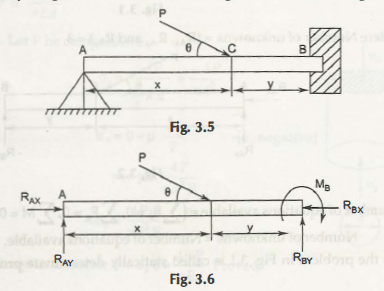Statics equations are available = 3

Number of unknowns = 5 = RBx, RBy, RAx, RAy‘and MB.

Now the degree of indeterminacy can easily be evaluated as

Degree of indeterminacy = 5-3 = 2

Solutions related to the problems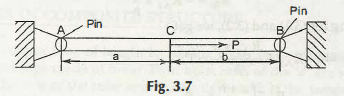If this figure is the problem, that you need to solve.

Now, go through carefully and you will get that Unknown numbers = 4

Avilable equations = 3

Degree of determinacy = 4-3 =1

By neglecting the bar’s weight the RAy addition of vertical reactions will be zero.

Now, consider the give case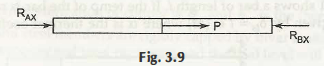Σ fx = 0  =>

RAX + P – RBX = 0

RAX – RBX = – P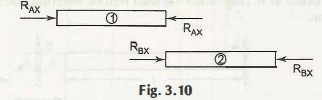Here it is important to find out another equation.

Elastic deformation for bar ACB

Two supports = Perfectly rigid (Assumed)

The total deformation for the total length (a + b) = zero.

δ1 = -RAx x a / AE

δ2 = – RBx x b / AE

δ1 + δ2 = 0

RAx x a + RBx x b = 0

RBx = – RAx x a /b

Now, after getting through both equations

RAx x a / b = p

RAx ( a +b / b) = p

RAx = pb / a+b

RBx x a /b = – pa / a +b

It means the exact direction of R ax will be completely opposite to the direction of P.

After understanding this you can easily solve the different problems.

Links of Next Mechanical Engineering Topics:-### Customer Reviews

My Homework Help
Rated 5.0 out of 5 based on 510 customer reviews at